## MP Board Class 6th Maths Solutions Chapter 14 Practical Geometry Ex 14.1

Question 1.
Draw a circle of radius 3.2 cm.
Solution:
Steps of construction :
(i) Mark a point ‘O’ with a sharp pencil where we want the centre of the circle.
(ii) Open the compasses for the required radius of 3.2 cm.
(iii) Place the pointer of compasses on O.
(iv) Turn the compasses slowly to draw the circle.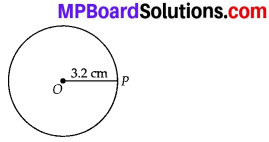Question 2.
With the same centre O, draw two circles of radii 4 cm and 2.5 cm.
Solution:
Steps of construction :
(i) Mark a point ‘O’ with a sharp pencil where we want the centre of the circle.
(ii) Open the compasses for 4 cm.
(iii) Place the pointer of the compasses on O.
(iv) Turn the compasses slowly to draw the circle.
(v) Again open the compasses for 2.5 cm and place the pointer of the compasses on O.
(vi) Turn the compasses slowly to draw the second circle.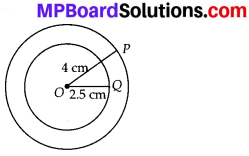Question 3.
Draw a circle and any two of its diameters. If you join the ends of these diameters, what is the figure obtained? What figure is obtained if the diameters are perpendicular to each other? How do you check your answer?
Solution:
(i) Mark a point ‘O’ with sharp pencil where we want the centre of the circle.
(ii) Place the pointer of the compasses on ‘O’.
(iii) Turn the compasses slowly to draw the circle.
(a) By joining the ends of two diameters, we get a rectangle. By measuring, we find AB = CD and BC = AD, i.e., pairs of opposite sides are equal and also ∠A = ∠B = ∠C = ∠D = 90°, i.e., each angle is of 90°.
Hence, it is a rectangle.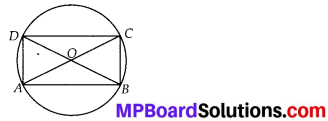(b) If the diameters are perpendicular to each other, then by joining the ends of two diameters, we get a square.
By measuring, we find that AB = BC = CD = DA, i.e., all four sides are equal. Also ∠A = ∠B = ∠C = ∠D = 90°, i.e. ,each angle is 90°.
Hence, it is a square.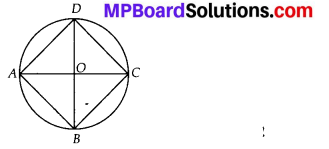Question 4.
Draw any circle and mark points A, B and C such that
(a) A is on the circle.
(b) 6 is in the interior of the circle.
(c) C is in the exterior of the circle.
Solution:
(i) Mark a point ‘O’ with sharp pencil where we want centre of the circle.
(ii) ‘Place the pointer of the compasses on ‘O’.
(iii) Turn the compasses slowly to draw a circle.
(a) Point A is on the circle.
(b) Point B is in the interior of the circle.
(c) Point C is in the exterior of the circle.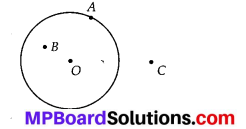Question 5.
Let A, B be the centres of two circles of equal radii; draw them so that each one of them passes through the centre of the other. Let them intersect at C and D. Examine whether $$\overline{A B}$$ and $$\overline{C D}$$ are at right angles.
Solution:
Steps of construction:
(i) Mark a point ‘A’ with sharp pencil.
(ii) Place the pointer of the compasses on A
(iii) Turn the compasses slowly to draw a circle with centre A.
(iv) Take a point ‘B’ on the circle with centre A.
(v) Place the pointer of the compasses on B.
(vi) Draw the circle with the radius same as radius of circle with centre A.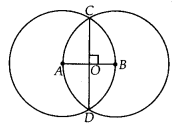Now, both circles intersect at C and D.
Let AB and CD intersect at O.
Now, measuring ∠COB, we get
∠COB = 90°
∴ $$\overline{A B}$$ and $$\overline{C D}$$ are perpendicular.